# Time of Flight Formula

Time of Flight Formula

A projectile is an object that is given an initial velocity, and is acted on by gravity. The amount of time it spends in the air is called the time of flight. If the ground from which the projectile is launched is level, the time of flight only depends on the initial velocity v0, the launch angle θ, and the acceleration due to gravity. The unit for the time of flight is seconds (s).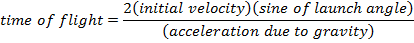t = time of flight (s)

v0 = initial velocity (m/s)

g = acceleration due to gravity (9.80 m/s2)

θ = angle of the initial velocity from the horizontal plane (radians or degrees)

Time of Flight Formula Questions:

1) A cricket jumps from one blade of grass to another. The cricket leaves the first blade of grass at an angle of 36.9°, at a velocity of 2.10 m/s. What is the cricket's time of flight?

Answer: The time of flight of the cricket can be found using the formula: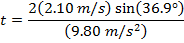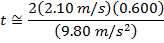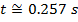The time of flight of the cricket is 0.257 seconds.

2) An athlete throws a javelin, which leaves her hand at an angle of 32.0°. If the javelin remains in the air for 3.20 s, what was the velocity of the javelin when it left the athlete's hand?

Answer: The initial velocity of the javelin can be found by rearranging the time of flight formula: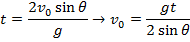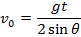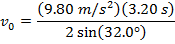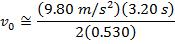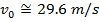The velocity of the javelin when it left the athlete's hand was 29.6 m/s.